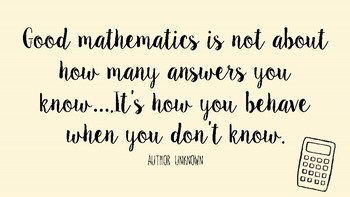# Mathematics

As Mathematicians…

Our children will:

• Become fluent in the fundamentals of mathematics, so that pupils develop conceptual understanding and the ability to recall and apply knowledge rapidly and accurately.
• Reason mathematically by following a line of enquiry, conjecturing relationships and generalisations, and developing an argument, justification or proof using mathematical language
• Solve problems by applying their mathematics to a variety of routine and non-routine problems with increasing sophistication, including breaking down problems into a series of simpler steps and persevering in seeking solutions